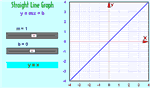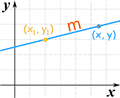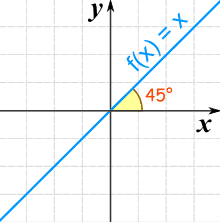# Linear Equations

A linear equation is an equation for a straight line

### These are all linear equations:y = 2x + 15x = 6 + 3yy/2 = 3 − x

Let us look more closely at one example:

### Example: y = 2x + 1 is a linear equation:The graph of y = 2x+1 is a straight line

• When x increases, y increases twice as fast, so we need 2x
• When x is 0, y is already 1. So +1 is also needed
• And so: y = 2x + 1

Here are some example values:

x y = 2x + 1
-1 y = 2 × (-1) + 1 = -1
0 y = 2 × 0 + 1 = 1
1 y = 2 × 1 + 1 = 3
2 y = 2 × 2 + 1 = 5

Check for yourself that those points are part of the line above!

## Different Forms

There are many ways of writing linear equations, but they usually have constants (like "2" or "c") and must have simple variables (like "x" or "y").

### Examples: These are linear equations:y = 3x − 6y − 2 = 3(x + 1)y + 2x − 2 = 05x = 6y/2 = 3

But the variables (like "x" or "y") in Linear Equations do NOT have:

### Examples: These are NOT linear equations:y2 − 2 = 03√x − y = 6x3/2 = 16

## Slope-Intercept Form

The most common form is the slope-intercept equation of a straight line:Slope (or Gradient) Y Intercept

### Example: y = 2x + 1

• Slope: m = 2
• Intercept: b = 1### Play With It !

You can see the effect of different values of m and b at Explore the Straight Line Graph

## Point-Slope Form

Another common one is the Point-Slope Form of the equation of a straight line:

 y − y1 = m(x − x1)### Example: y − 3 = (¼)(x − 2)

It is in the form y − y1 = m(x − x1) where:

• y1 = 3
• m = ¼
• x1 = 2

## General Form

And there is also the General Form of the equation of a straight line:

 Ax + By + C = 0 (A and B cannot both be 0)

### Example: 3x + 2y − 4 = 0

It is in the form Ax + By + C = 0 where:

• A = 3
• B = 2
• C = −4

There are other, less common forms as well.

## As a Function

Sometimes a linear equation is written as a function, with f(x) instead of y:

 y = 2x − 3 f(x) = 2x − 3 These are the same!

And functions are not always written using f(x):

 y = 2x − 3 w(u) = 2u − 3 h(z) = 2z − 3 These are also the same!

## The Identity Function

There is a special linear function called the "Identity Function":

f(x) = x

And here is its graph:It makes a 45° (its slope is 1)

It is called "Identity" because what comes out is identical to what goes in:

In Out
0 0
5 5
−2 −2
...etc ...etc

## Constant Functions

Another special type of linear function is the Constant Function ... it is a horizontal line:f(x) = C

No matter what value of "x", f(x) is always equal to some constant value.

## Using Linear Equations

You may like to read some of the things you can do with lines: